# C++ Program to Check Vowel or Consonant

This article will teach you how to use C++ to determine whether the given alphabet is a vowel or a consonant. The program is created in the following ways:

Important: If the pronunciation of a character is produced by humans, the breath flows out of the mouth without being blocked by teeth, lips, or tongue. Then the character is called a vowel.

### List of Vowels

The vowels are a, e, i, o, u, or A, E, I, O, U.Based on these five vowels, we developed a program that determines whether a user-input character is a vowel or not at run-time.

Note: Sometimes, y can also be called a vowel.

As already mentioned, we'll only deal with the five vowels listed above. We won't go into detail about vowels because we only need to create the program on them. So let's move on to the program.

## In C++, check if an alphabet is a vowel or consonant using the if-else

To check whether the input alphabet is a vowel or not (consonant) in C++ programming, you have to ask the user to enter an alphabet first, then check and print the message as shown in the program given below:

If the input alphabet is equal to any of the following 10 characters (5 lowercase vowels and 5 uppercase vowels):

• a, A, e, E, i, I, o, O, u, U

It is called a vowel. Here is the program:

```#include<iostream>
using namespace std;
int main()
{
char ch;
cout<<"Enter an Alphabet: ";
cin>>ch;
if(ch=='a' || ch=='e' || ch=='i' || ch=='o' || ch=='u')
cout<<"\nIt is a Vowel";
else if(ch=='A' || ch=='E' || ch=='I' || ch=='O' || ch=='U')
cout<<"\nIt is a Vowel";
else
cout<<"\nIt is a Consonant";
cout<<endl;
return 0;
}```

This program was built and runs under the Code::Blocks IDE. Here is its sample run: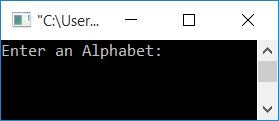Now supply any alphabet, say U, and press the ENTER key to see the following output: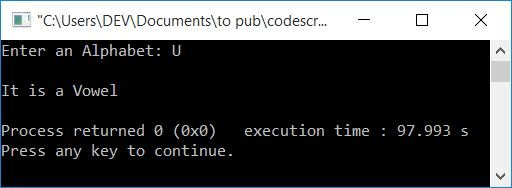Here is another sample run with user input as c: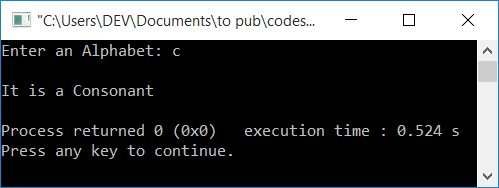This program has a limitation: if the user enters 4, then the output produced by the above program is "It is a consonant." But 4 is not a consonant; rather, it is a number. Therefore, we have another program to deal with this type of input.

### What if the user enters neither a vowel nor a consonant?

The program given below is the solution to user input that is neither vowel nor consonant:

```#include<iostream>
using namespace std;
int main()
{
char ch;
cout<<"Enter the Character: ";
cin>>ch;
if((ch>='a' && ch<='z') || (ch>='A' && ch<='Z'))
{
int lowerVowel, upperVowel;
lowerVowel = (ch=='a' || ch=='e' || ch=='i' || ch=='o' || ch=='u');
upperVowel = (ch=='A' || ch=='E' || ch=='I' || ch=='O' || ch=='U');
if(lowerVowel)
cout<<"\nIt is a lowercase Vowel";
else if(upperVowel)
cout<<"\nIt is an uppercase Vowel";
else
cout<<"\nIt is a Consonant";
}
else
cout<<"\nIt is neither Vowel nor Consonant";
cout<<endl;
return 0;
}```

Here is an example of a run with user input as 4: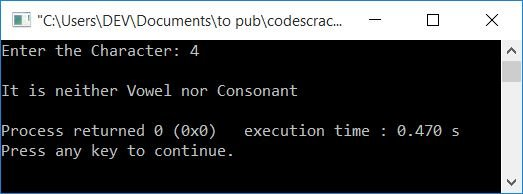And here is another sample run with user input as E: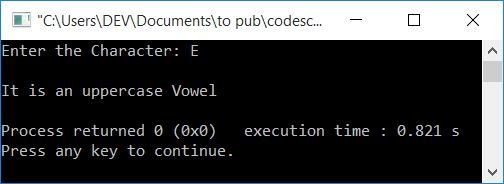The statement,

`lowerVowel = (ch=='a' || ch=='e' || ch=='i' || ch=='o' || ch=='u');`

initializes 1 to lowerVowel if the value of ch gets matched to any of the characters a, e, i, o, or u. Because 1 is a condition that the if block treats as true, program flow is directed inside the if's body if lowerVowel remains at 1. Otherwise, in a similar way, the if-else block gets executed. And if both the lowerVowel and upperVowel do not retain their value of 1, else's body is executed, and a message is printed; "It is a Consonant."

## Using the switch, check the vowels

This is the last program that uses switch case to check and print whether an input character is a vowel or a consonant:

```#include<iostream>
using namespace std;
int main()
{
char ch;
cout<<"Enter an Alphabet: ";
cin>>ch;
switch(ch)
{
case 'a':
case 'A':
case 'e':
case 'E':
case 'i':
case 'I':
case 'o':
case 'O':
case 'u':
case 'U':
cout<<"\nIt is a Vowel";
break;
default:
cout<<"\nIt is a Consonant";
break;
}
cout<<endl;
return 0;
}```

It will produce the same output as the very first program in this article.

#### The same program in different languages

You might enjoy the following program in another programming language:

C++ Quiz

« Previous Program Next Program »